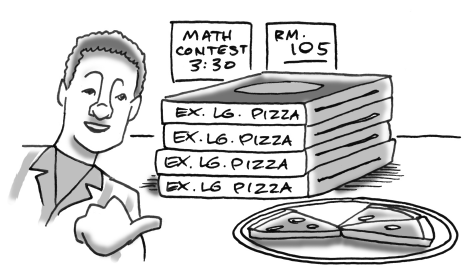### Home > CC3MN > Chapter 6 Unit 10 > Lesson INT1: 6.4.2 > Problem6-145

6-145.

After the math contest, Basil noticed that there were four extra-large pizzas that were left untouched. In addition, another three slices of pizza were uneaten. If there were a total of $51$ slices of pizza left, how many slices does an extra-large pizza have?

Begin by defining a variable. Let $x =$ the number of slices in one pizza

Write an equation that says that the slices in $4$ pizzas plus the $3$ extra slices equals $51$ total slices.